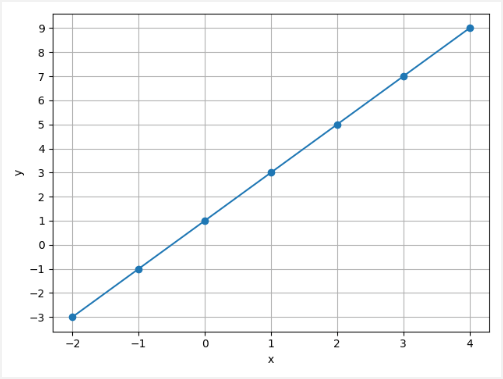# Simple Linear Regression using PyTorch

Simple linear regression is a statistical method that is used to analyze the relationship between two continuous variables:

• `x` - independent variable also known as explanatory or predictor.
• `y` - dependent variable also known as response or outcome.

Let's say we have the following sets of numbers:

You may notice that the `x` value is increasing by 1, and the corresponding `y` value is increasing by 2. So relationship is `y = 2 * x + 1`.This tutorial provides example how to create and train a model which predicts the value of `y` for the given value of `x` using PyTorch.

We define arrays of `x` and `y` values for training a model. Model contains one layer. It has one input and one output.

We choose the mean squared error (MSE) as loss function and stochastic gradient descent (SGD) as optimizer. A learning rate is 0.01.

During training we perform forward pass to compute predicted `y` values by using `x` values. The loss function is used to compute error between the predicted and true values of `y`. After that, we explicitly set the gradients to zero, perform backward pass and use optimizer to update the network weights. Training is performed 400 epochs.

``````import torch

xs = torch.Tensor([[-2.0], [-1.0], [0.0], [1.0], [2.0], [3.0], [4.0]])
ys = torch.Tensor([[-3.0], [-1.0], [1.0], [3.0], [5.0], [7.0], [9.0]])

model = torch.nn.Sequential(
torch.nn.Linear(1, 1)
)

loss_fn = torch.nn.MSELoss()
optimizer = torch.optim.SGD(model.parameters(), lr=0.01)

epochs=400
for epoch in range(epochs):
ys_pred = model(xs)

loss = loss_fn(ys_pred, ys)

loss.backward()
optimizer.step()

x = torch.Tensor([[15.0]])
y = model(x).item()
print(y)``````

When the training is completed, we try to predict value of `y` for a previously unknown value of `x`. In our case, trained model returns `y` equal to 31.001181 when `x` is 15.0. We can verify:

``y = 2 * x + 1 = 2 * 15 + 1 = 31``